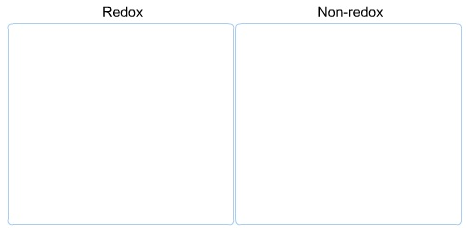# Problem: Classify each of the following as a redox reaction or a non-redox reaction. 2CO + O2 → 2CO2 SO3 + H2O →H2SO4 Zn + CuCl2 → ZnCl2 + Cu HCl + NaOH → H2O + NaCl

###### FREE Expert Solution

To classify each of the following as a redox reaction or a non-redox reaction:

For Redox reactions, oxidation and reduction both occur in a reaction:

Recall the mnemonics LEO GER.

Lose               Gain

Electron         Electrons

Oxidation       Reduction

Step 1: Determine oxidation state:

2CO + O2 → 2CO2

• O.S. of O = -2 (CO, CO2 are not peroxides)
• O.S. of O in O20 (natural state/elemental form)
• neutral compound → sum of O.S. = 0
• For CO:
• O.S. of C + O.S. of O = 0
• O.S. of C + -2 = 0
• O.S. of C = +2
• For CO2
• O.S. of C +2( O.S. of O) = 0
• O.S. of C + 2(-2) = 0
• O.S. of C = +4

Step 2: Changes in O.S.:

C:        +4  +4         increased/lost electrons oxidation

O:         –2           decreased/gained electrons reduction

100% (141 ratings)###### Problem Details

Classify each of the following as a redox reaction or a non-redox reaction.2CO + O2 → 2CO2

SO3 + H2O →H2SO4

Zn + CuCl2 → ZnCl2 + Cu

HCl + NaOH → H2O + NaCl